Chemical equilibrium (2011 VCE) 1) Consider the following equilibrium expression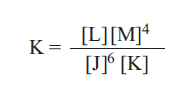The equation for the forward reaction is: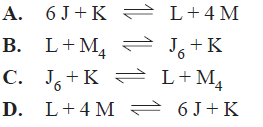Solution. 2) Consider the following equilibrium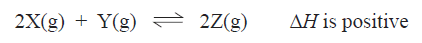A catalyst is added to the mixture at time t. The volume and temperature of the mixture remain constant. Which of the following graphs best represents the change in the ratio [Z]/[X]?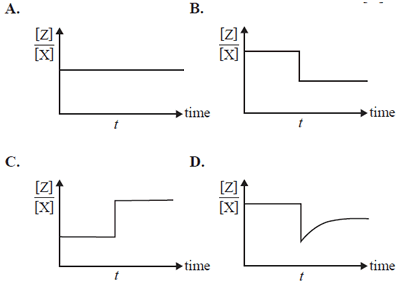Solution3) Hydrogen and fluorine react according to the following equation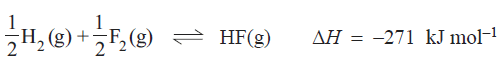In an experiment 0.200 mol of hydrogen and 0.340 mol of fluorine were placed in a reaction vessel of volume V litres Once equilibrium was established there was 0.220 mol of HF present in the reaction vessel. Which of the following expressions can be used to calculate the equilibrium expression for this reaction.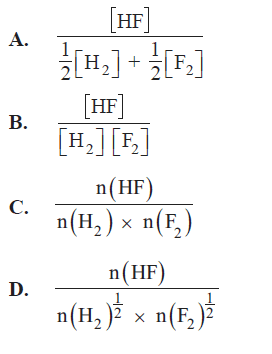Solution4) The reaction in question 3) above is placed in a reaction chamber surrounded by a heat exchanger that keeps the reaction temperature constant. The amount of heat absorbed by the heat exchanger in order to keep the temperature constant is: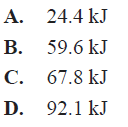Solution5) It is proposed to indirectly determine the concentration of Fe3+ ions in a solution by using UV-visible spectroscopy to measure the concentration of red coloured FeSCN2+ ions.generated by the equilibrium reaction.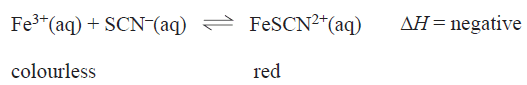This procedure would provide the most accurate estimate of Fe3+ ions in the original solution if: a) the value of the equilibrium constant is small, an excess of SCN- is used and the analysis is carried out at a low temperature b) the value of the equilibrium constant is large, an excess of Fe3+is used and the analysis is carried out at a high temperature c) the value of the equilibrium constant is small, an excess of Fe3+is used and the analysis is carried out at a high temperature d) the value of the equilibrium constant is large, an excess of SCN- is used and the analysis is carried out at a low temperature Solution6) Nitrogen oxides are commony found in the atmosphere in areas where there is serious atmospheric pollution. Nitrogen monoxide is generated from the reaction between nitrogen and oxygen.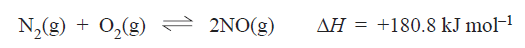A sealed container is filled with 1.00 mole of NO(g) and equiibrium is established. The temperature is maintained at 1500 oC. a) Explain why the rate of reaction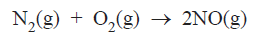will never be greater than the rate of the reaction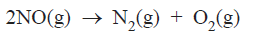i) while the system is reaching equilibrium. Solution Click for examiner's comments ii) once equilibrium is estabished. Solutionb) NO(g) is produced in combustion engines such as a car engine.Catalysts based on platinum and palladium are used in the exhaust system to decompose NO(g) into N2 (g) and O2 (g). i) Based on the information provided, explain why combustion engines are such good chemical environments for the production of NO(g). Solution ii) Sketch, on the axes provided below, a fully labelled energy profile diagram for the decomposition reaction of NO. Indicate on the diagram the effect of using a catalyst in this reaction.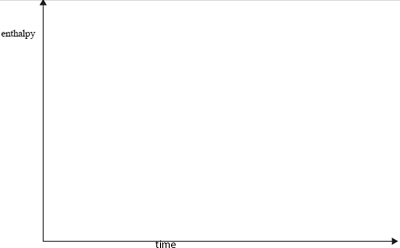Solution7) Methanol is produced on an industrial scale by the catalytic conversion of a mixture of hydrogen and carbon monoxide gases at a temperature of 520 K and a pressure of 50 to 100 atmopsheres. The reaction that occurs in the methanol converter is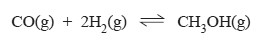Carbon monoxide gas and hydrogen gas are mixed in a reaction vessel and equilibrium is established. The graph below shows how the concentration of methanol in this vessel changes with time at three different temperatures. The pressure is the same at each temperature.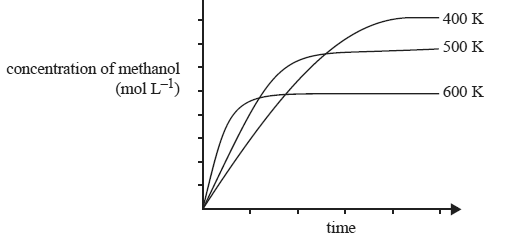i) Is the reaction exothermic or endothermic. Explain Solution ii) Explain why a moderately high temperature of 520 K is used although the equilibrium concentration of methanol is greater at lower temperatures. Solution iii) Explain why, at a given temperature, the use of high pressures results in a greater equilibrium concentration of methanol. Solution iv) A catalyst consisting of a mixture of copper, zinc and aluminium is used to increase the rate of this reaction. Explain how a catalyst can increase reaction rate. Solution